Next: Power-Law Expansion Up: Scale Factor Evolution Previous: The Scale Factor Equation

## Correcting for Staggered Leapfrog

In practice the program uses a staggered leapfrog algorithm so in solving forthe value of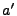is known at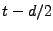where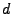is the time step. See section 6.4 for more details. The solution to this problem is to use the two equations(6.27)

whereandrefer to the values ofatandrespectively and all other variables are evaluated at time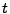. Take the evolution equation to be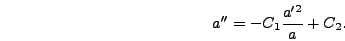(6.28)

Plugging this form into equation (6.27) and eliminatinggives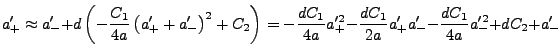(6.29)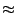(6.30)(6.31)

To determine whether to use the plus or minus sign in equation (6.31) consider the limit as. In this limit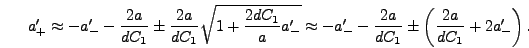(6.32)

This suggests that the plus sign must be used in order to reduce to the limit. Hence(6.33)

In the program it's useful to calculate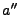, which is roughly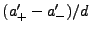, so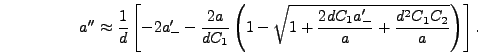(6.34)

Thus equation (6.26) becomes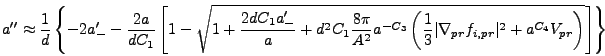(6.35)

where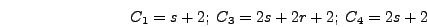(6.36)Next: Power-Law Expansion Up: Scale Factor Evolution Previous: The Scale Factor Equation